# Top 10 Machine Learning Algorithms that will make work simple## WHAT IS MACHINE LEARNING?

Machine learning is defined as a  scientific study of statistical models, computer systems and algorithms that help do the work without any supervision. This branch of study is a subset of artificial intelligence and uses patterns and inferences from similar situations to complete the task. These algorithms are built using sample data which is known as the training data. This training data helps in making predictions, which helps the system to perform the task without being explicitly telling it what is to be done.

For example, Email Filtering is a process of grouping emails that hit your inbox by analyzing and organizing it based on specific criteria. Computer Vision is a scientific division that allows computers to achcess images and videos and gain insights into a human vision. Both of these fields use machine learning algorithms extensively.

Although Machine learning is closely coupled with computational statistics, it focuses on mathematical optimization to make predictions. This helps in computing and delivering various theories and application fields for the area in which it is used. Data Mining is also a part of machine learning that deals with exploratory analysis of data mostly done without supervision. Machine learning is often confused with Artificial Intelligence and Deep Learning. The below image draws a line of difference between these three concepts.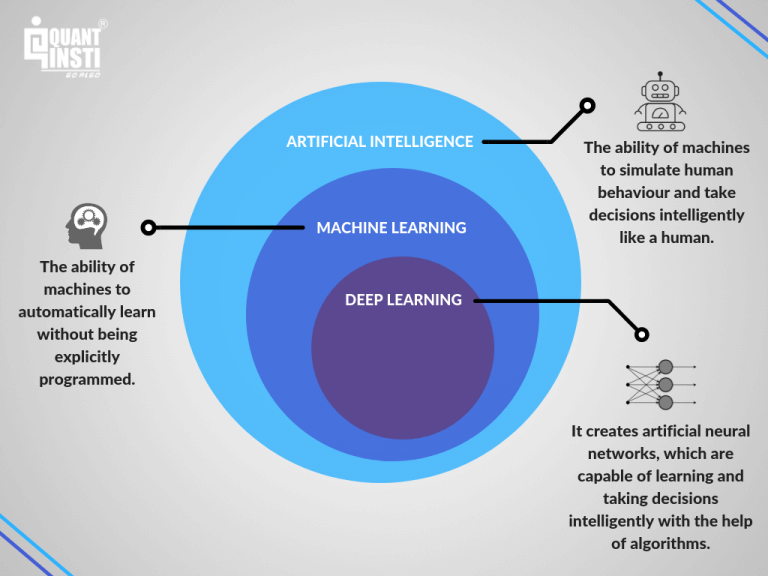Jump straight to the section of the post you want to read:

TYPES OF MACHINE LEARNING

Machine learning can be broadly divided into 4 categories.

1. SUPERVISED LEARNING

It is built using a mathematical model and has data pertaining to both the input and the output. For instance, if the goal is to find out whether a certain image contained a train, then different images with and without a train will be labeled and fed in as training data.

So, basically, you have the inputs ‘A’ and the Output ‘Z’. Supervised learning uses a function to map the input to get the desired output.

Z=f(A)

In order to solve an issue using Supervised learning, the following steps must be followed:

1 . Analyze the training data.

1. Gather the input and output sample for analysis
2. Figure the input representation and the learned function. The input information should not be extensive but must contain enough data to interpret and predict the output
3. Determine the structure that you are going to use for the learned function.
4. Complete the design structure and cross-validate
5. Evaluate the accuracy and test the function using a different set.

These algorithms are useful in classification and regression problems. These problems aid in time series prediction.

1. Classification: In a classification problem the output variable belongs to some category. For example: red, blue, disease, no disease.
2. Regression: A regression problem is where the output has a value. For example: Dollars, Kilogram

Some of the most popular algorithms under supervised learning includes:

1 . Support Vector Machines

1. linear regression
2. logistic regression
3. naive Bayes
4. linear discriminant analysis
5. decision trees
6. k-nearest neighbor algorithm
7. Neural Networks (Multilayer perceptron)
8. Similarity learning
9. Semi-Supervised Learning

This is similar to Supervised learning but the images fed as training data will be incomplete. For instance, there will be images with trains that may not be labeled.  It will have a limited set of values.

Semi-supervised data is a way how most humans learn. Let’s say the father points to his daughter and says “That brown color animal is a Dog”. The child knows the animal, but it interprets the nature of the animal by observing it.

Semi-supervised Learning is done based on 3 assumptions.

1. Continuity assumption: Points next to each other are likely to be alike.
2. Cluster assumption: Data is assumed to be in discrete clusters. And, each cluster has a common label.
3. Manifold assumption: Data lies on a much lower dimension than the input in a mainfold.
4. UNSUPERVISED LEARNING

Here the training data fed into the system will only have input values. The output images will not be labeled. This is used to find the structure of data points. It is used to identify patterns among data points.

Here you only have the input data (A) and no output data (Z). But, this model helps you identify the underlying structure or in other words the distribution in the data which helps in learning more about the data. The major application of unsupervised learning lies in the field of density estimation.

These algorithms can be grouped into clustering and association problems.

1. Clustering: A clustering problem allows you to discover inherent groupings in the data. For Example: Buyer Behaviour
2. Association:  An association rule allows you to categorize people based on a action. For Example: People who like Y will also like Z.

Popular Algorithms in unsupervised learning:

1. hierarchical clustering
2. k-means
3. mixture models
4. DBSCAN
5. OPTICS algorithm
6. ACTIVE LEARNING

Here the training data has the desired output labels but are provided with limited input labels. For instance, lets say ‘T’ is the total set of all data under consideration. T should be broken into the following subsets.

T K, I is the known data points

T U,I is the unknown data points

T C,I is the subset of data chosen from T U,I

TOP 10 MACHINE LEARNING ALGORITHMS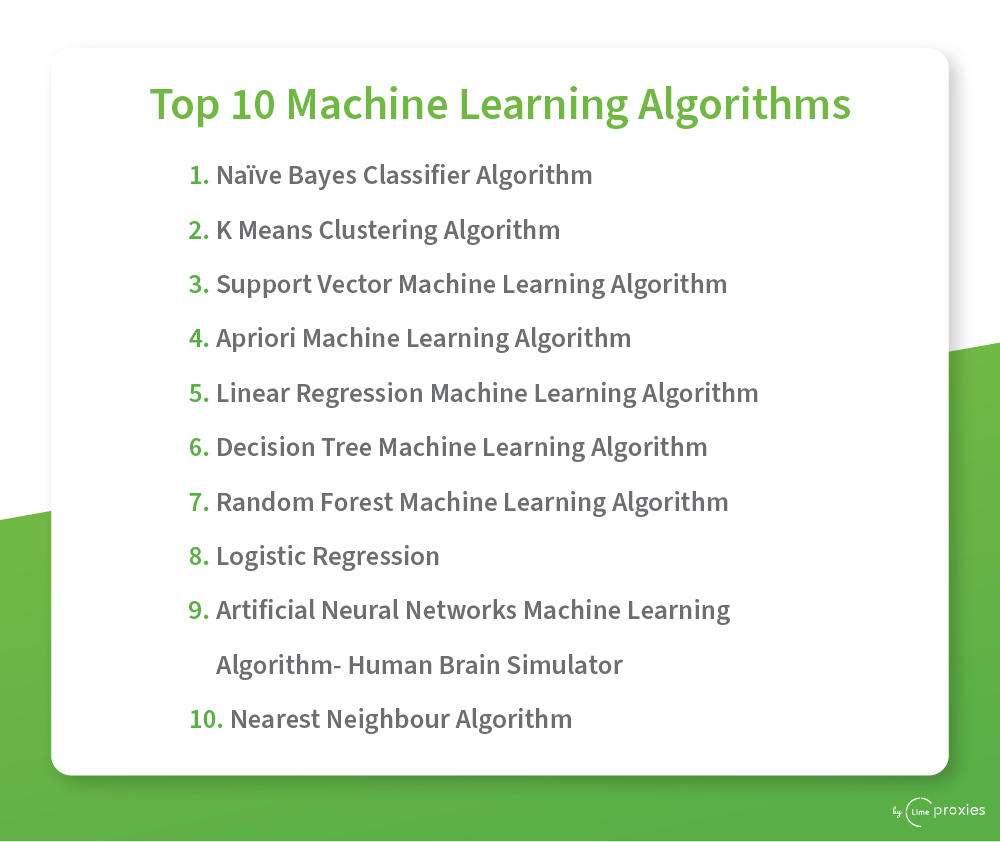## 1) NAÏVE BAYES CLASSIFIER ALGORITHM

Naive Bayes Classifier algorithm helps in classifying the web page, email or any lengthy content. Spam filtering in the email is a popular example of this algorithm, The Spam filter classifies if the email is a Spam or Not Spam.

This algorithm is one of the most common learning methods used to group similarities.

WHEN TO USE NAÏVE BAYES CLASSIFIER?

1. When your data set is medium to large.
2. When there are multiple attributes to the instance.

APPLICATIONS OF NAÏVE BAYES CLASSIFIER

1 . Sentiment Analysis- Used to identify if the response from the audience is positive or negative

1. Document Categorization- Page ranking mechanism by Google. It is also used to classify articles based on Tech, sports etc.
2. Email Spam Filtering- To check if the emails are spam or not

1. Performs exceptionally well for categorical data
2. Converges faster even with less training data
3. It works extremely well for data prediction

2) K MEANS CLUSTERING ALGORITHM

K-means is based on unsupervised learning of cluster analysis. It is a non-descriptive iterative method and operates on a given data set. There are a number of predefined clusters called k.  The output of this algorithm is nothing but the k clusters with input data partitioned among different clusters.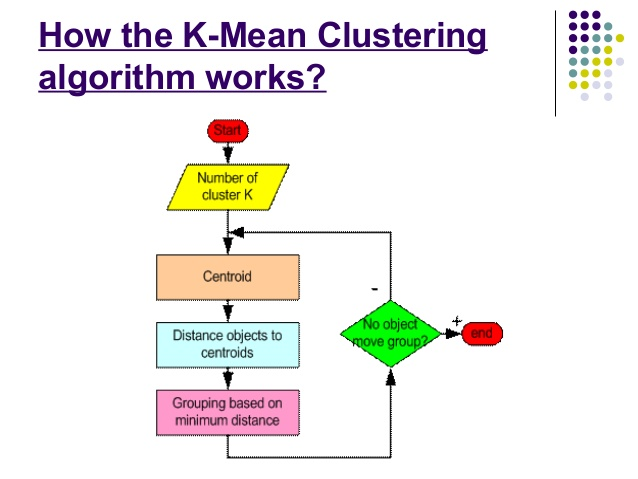Source-slideshare

1. When used for globular clusters, K-Means produces tighter clusters
2. Even when a small value of K is provided, clustering is faster

Applications of K-Means Clustering

1 . It is used by different search engines to determine the relevance rates. It helps in reducing the computational time.

1. Data Science libraries implement K means

## 3) SUPPORT VECTOR MACHINE LEARNING ALGORITHM

Support Vector Machine is part of supervised machine learning.

The algorithm is used in regression problems. Here the data set is fed into Support Vector Machine and the algorithm studies the classes, this helps it to classify new data.

Since there are too many hyperplanes, the algorithm tries to maximize the distance between the classes. When the line that maximizes the distance is found, there is a high probability to generalize the unseen data.

There are two categories of Support Vector Machine Learning Language:

1 . Linear Support Vector Machine Learning Language – In linear Support Vector Machine Learning Language the training data is separated using a hyperplane.

1. Non-Linear Support Vector Machine Learning Language- In non-linear Support Vector Machine Learning Language, you cannot separate the training data with the help of a hyperplane.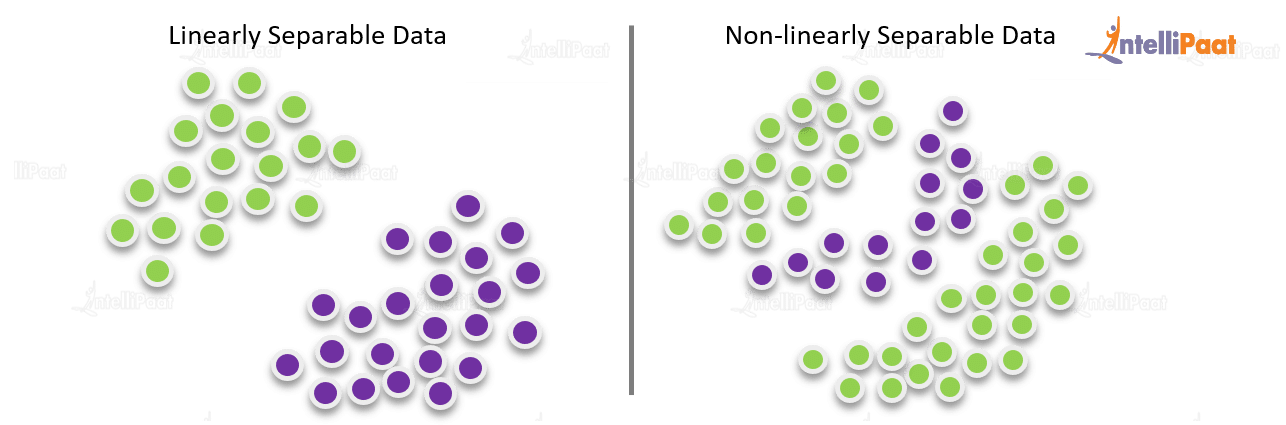Source-intellipaat

1 . It is extremely accurate

1. Prediction of future data works great

APPLICATIONS OF SUPPORT VECTOR MACHINE

1 .Support Vector Machine Learning Language is used for stock market forecasting to compare the relative forecast

1. Data Science Libraries in Python
2. Data Science Libraries in R

## 4) APRIORI MACHINE LEARNING ALGORITHM

Apriori algorithm is also developed based on the unsupervised machine learning algorithm. It generates an association for the given data set. It is based on a rule that if something occurs one cluster then there is a high probability that another item happening to that cluster will have the same probability of happening. The rules are written in an  If and Then format.

The rule of the algorithm is that if an object occurs frequently then its subsets will also follow the same pattern. If the object is infrequent, then its subsets will be infrequent.

1 . Easy to implement.

1. Helps manage large sets of data

APPLICATIONS OF APRIORI ALGORITHM

1 . Detecting Drug Reactions

2. Auto-Complete Applications

## 5) LINEAR REGRESSION MACHINE LEARNING ALGORITHM

Linear Regression algorithm shows how two variables can be coupled together and how change in one variable affects the other.

1 . Requires minimal tunning

1. Extremely fast

APPLICATIONS OF LINEAR REGRESSION

1 . Sales Estimation

1. Assessment of Risks

## 6) DECISION TREE MACHINE LEARNING ALGORITHM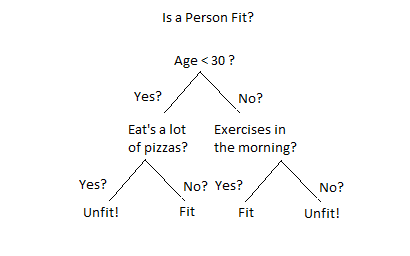Source- Eckovation

Decision Tree algorithm is similar to how humans make decisions. For instance, let us say that you want to buy a car and you ask your friend for a suggestion. You give a list of cars that you have researched to your friend and then your friend asks you the main features that you are looking for, he does some research and then adds that to the list. When all the research is done, you will decide the vehicle that you’d want to buy.

It is a representation of all the possible outcomes based on the set of conditions.

TYPES OF DECISION TREES

1 . Classification Trees- The data set in these trees are categorized into different classes based on the response.

1. Regression Trees- Here the response and target follow a regression pattern and is used in prediction analysis.

## WHY USE THE DECISION TREE MACHINE LEARNING ALGORITHM?

1 . It helps in making decisions during the time of uncertainty.

1. Helps data scientists identify how the course of the application would have changed if a different decision was taken.
2. It helps in fast-forwarding predictions and backtracking decisions.

Interesting Read : 50 Reporting Tools for Data Scientists in 2019 From Experts

1 . Easy to understand even for someone with a nontechnical background.

1. Can handle both categorical and numerical values which means the data set is not a constraint.
2. It can even be used during instances where the data is non-linerly related.
3. Useful for data exploration
4. It helps in saving data preparation time as it is not sensitive to the data.

APPLICATIONS OF DECISION TREE MACHINE LEARNING ALGORITHM

1. Used in the Finance department for option pricing.
2. Remote Sensing
3. Bank Applications to find the history of payment
4. In Hospitals to find diseases and at-risk patients

## 7) RANDOM FOREST MACHINE LEARNING ALGORITHM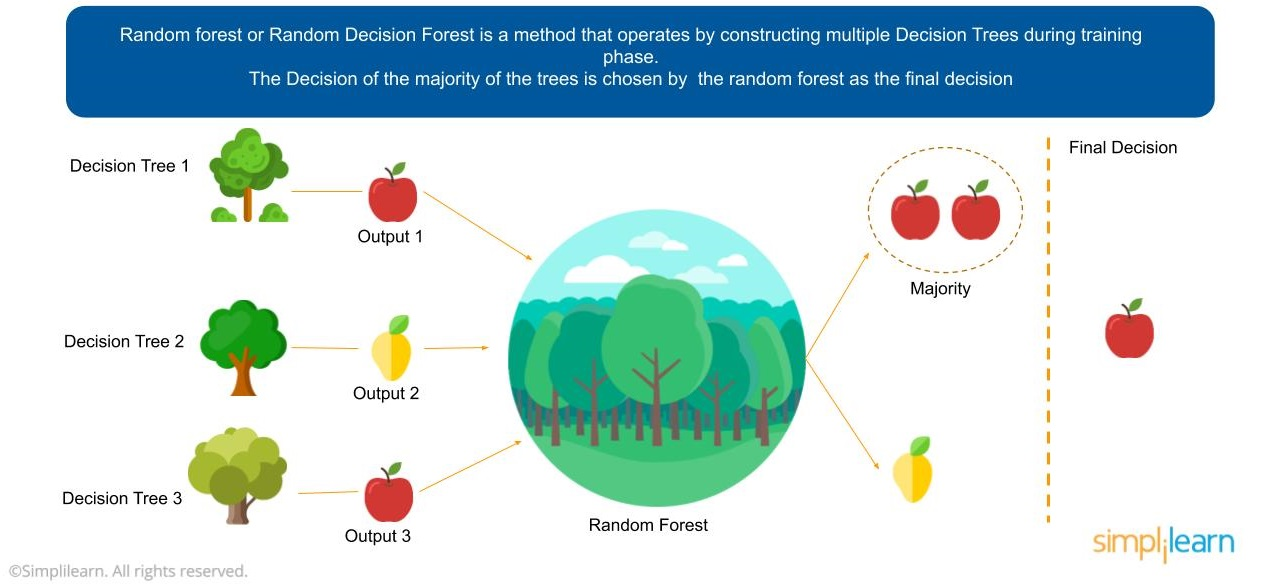Source-simplilearn

Random Forest uses an approach to create a bunch of decision trees. These decision trees have a random data subset. The algorithm is fed with sample data and different predictions are collected. In this method, the final output of all the decision trees are combined to decide the final output.

This goes by the majority. Let’s say if 7 friends are in a decision-making process of which 3 do not want to go to a restaurant by 4 does, then the decision will be made in favor of the 4.

1 . It is open source

1. It maintains accuracy when there is missing information
2. Easy to implement
3. Requires less preparation time
4. It helps in regression testing when there is too much noise.
5. Has a high satisfaction rate

APPLICATIONS OF RANDOM FOREST MACHINE LEARNING ALGORITHMS

1 . Banks to predict the risk of loan applicant

1. Automobile industry to find the probability breakdown of a part
2. Health care industry to indentify if the patients are susceptible to develop a chronic disease.
3. User interaction on social media

## 8) LOGISTIC REGRESSION

Logistic regression uses a linear combination of features to what the output would be.

For example, if you would like to know if there is a thunderstorm or not tomorrow you cannot rely on linear data to find this out. There can be only 2 possibilities here. 1. There could be a thunderstorm. 2. There cannot be one.

Logistic regression can be classified into 3 types –

1 . Binary Logistic Regression – This is used when there are only 2 possible outcomes for the problem - an yes or a no.

2. Multi-nominal Logistic Regression - when there are three or more possible responses. For example, the most used search engine in the US.

3. Ordinal Logistic Regression - When there is 3 or more categorical response to the data. For example ratings given by a customer for a product.

1 . Complexity is less.

1. Extremely robust as there is no need for the variables to have an equal distribution.

APPLICATIONS OF LOGISTIC REGRESSION

1 . Logistic regression algorithm is used in epidemiology. It helps in identifying risk factors of various diseases and lets them plan preventive measures accordingly.

1. Predict election
2. Weather prediction

## 9) ARTIFICIAL NEURAL NETWORKS MACHINE LEARNING ALGORITHM- HUMAN BRAIN SIMULATOR

Human brain is a complex thing. It keeps assimilating data from a lot of things such as the things that we see, hear, touch etc. All of this information is stored in the brain and when a similar situation or vision is seen again, it will pull up these details from the brain. Now, what if the same thing was done by a computer and not the brain. This is what this algorithm does. The complexity of the algorithm depends on the input and output fed into it. The algorithm is constantly evolving and cannot recognize things as fast as a human brain. But, with time, we will be there.

APPLICATIONS:

1. Text classification and categorization
2. Name Entity Recognition
3. Speach tagging

## 10) NEAREST NEIGHBOUR ALGORITHM

Nearest neighbor algorithm is the first of many to solve the traveling salesman problem effectively.

Traveling Salesman problem: Given a list of cities and the distances between each pair of cities, what is the shortest possible route that visits each city and returns to the origin city?The K-nearest neighbor algorithm is all about forming the major vote between K and similar observations. It is defined using the distance between two points. Here is a popular method that is used: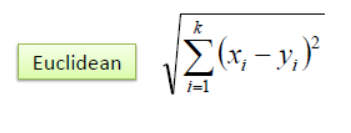1 . Easy  implementation

1. Flexible distance choices
2. Handles multi-class cases

APPLICATIONS:

1 . Measure documentation similarity

1. Pattern recognition
2. Gene expression
3. Find distance

Machine learning is a fast emerging field and has applications in almost every area of study. It is a quick way to resolve problems that are extremely difficult or time consuming. Hope you found this blog useful. Let us know your thoughts in the comments section below.Rachael Chapman

A Complete Gamer and a Tech Geek. Brings out all her thoughts and Love in Writing Techie Blogs.PrevHow to choose the best proxy for Price Intelligence?
NextProxy Lists: What Are They and What Are They Used for?